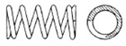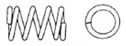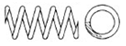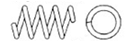# Compression Springs FAQ

What is the difference between the end types for compression spring?
There are four basis end types for compression springs. The chart below explains the differences.

Ends - closed and ground

Same as “closed not ground ends”, except that the end is ground to provide a flat plane.Ends - closed not ground

Ends of compression springs where the pitch of the end coils is reduced so that the end coils touch.Ends - open and ground

Same as “open not ground ends” followed by an end grinding operation.Ends - open not ground

End of a compression spring with a pitch for each coil (last coils not touching).What does it mean when a compression spring “takes a set”?

This is when a compression spring is slightly overstressed. When it is compressed beyond its maximum safe deflection, the spring becomes shorter (takes a set) than it was before it was compressed.

What is the solid height of a compression spring?

This is the length of the spring when it is compressed so all coils touch each other.

What is the difference between “pitch” and “space between coils” of a compression spring?

The “space between coils” is the actual distance from the top of one coil to the bottom of the next coil. Whereas the “pitch” is the distance from center of one coil to the center of the next coil. Mathematically, the “pitch” is ALWAYS one wire size larger than the “space between coils”.